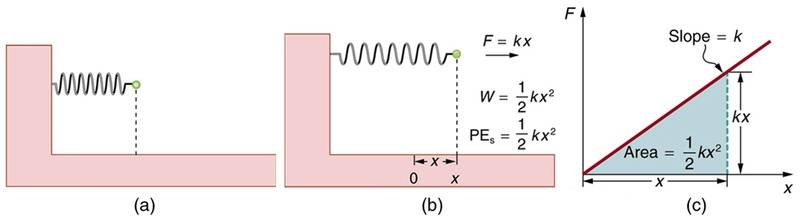# A cylinder with cross-section area A floats with its long axis vertical

Homework Helper
Gold Member
f = force
a = area
p = rho/density
g = gravity
x= displacement
Water acts like a compressing spring on your cylinder: the lower it is pushed, the higher is the resistive vertical force.
As area, density and gravity acceleration are all constant, we could make their product a constant k.
Then, we have a problem similar to the work done by, or on, a spring.

https://courses.lumenlearning.com/suny-physics/chapter/7-4-conservative-forces-and-potential-energy/•erobz
•